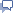# Database of Number Correlations

The purpose of this section is to discover unexpected connections between various material and occult phenomena. A great resource for mathematicians. Notable Events for over 2000 years of history: Discover unusual synchronicities in World History. 2000 plus pages.
 <54 Number Data-Base Random Number 56>

## The Number 55: Properties and Meanings

Prime Factors of 55=5x11.

55 can be Partitioned 28 times with each term no larger than 2.

55 can be Partitioned 280 times with each term no larger than 3.

55 can be Partitioned 1495 times with each term no larger than 4.

55 is a Fibonacci Number.

55 is a Triangular Number.

55 is a Heptagonal Number.

55 is a Centered Nonagonal Number.

55 is a Centered 18-gonal Number.

55 is a Square Pyramidal Number.

55 is the largest Triangular Number in the Fibonacci Sequence.

the sum of 5 successive Triangular Number (3+6+10+15+21 = 55)

Sum of the two series of following numbers: 1, 2, 4, 8 and 1, 3, 9, 27=55.

Sum of the first 5 Square Numbers = 1 + 4 + 9 + 16 + 25 = 55

Sum of the 8 factors of 36 = 1 + 2 + 3 + 4 + 6 + 9 + + 18 = 55

Caesium is a soft, silver-white, metallic element with atomic number 55. It is a very reactive element, exploding on contact with water and reacting instantly with air.

The Chemical Element Manganese has one Stable Isotope. It has 55 neutrons.

"The Ancient Chinese believed that "the sum total of heavenly numbers and earthly numbers is 55. It is this...which sets the gods and demons in movement."

In the year 55 AD King Nicander II of Hellas died. He was succeeded by Pausanias III.

In the year 55 AD King Vologeses of Parthia was killed in battle against the Kushans. He was succeeded by his son, who reigns as King Mithridates III.

 <54 Number Data-Base Random Number 56>Share any properties and meanings for particular Numbers...contact me directly, thanks.# Database of Number Correlations

The purpose of this section is to discover unexpected connections between various material and occult phenomena. A great resource for mathematicians. Notable Events for over 2000 years of history: Discover unusual synchronicities in World History. 2000 plus pages.